# (1 point) If the differential equation d2x m 7 dx dt + 8x = 0 dt2...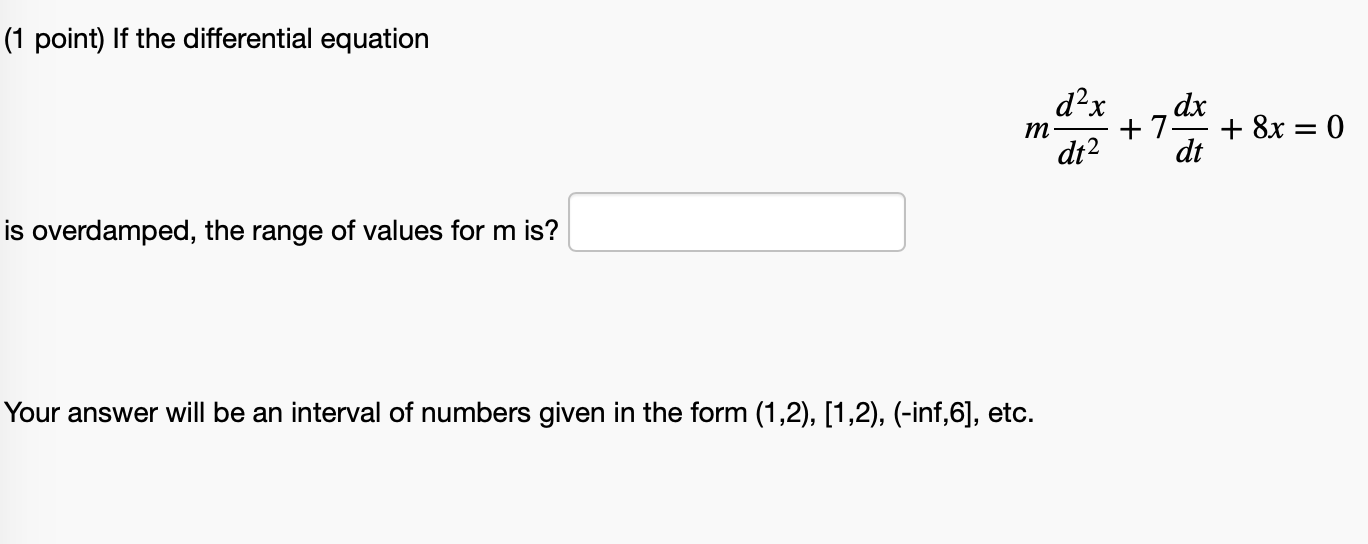(1 point) If the differential equation d2x m 7 dx dt + 8x = 0 dt2 is overdamped, the range of values for m is? Your answer will be an interval of numbers given in the form (1,2), (1,2), (-inf,6], etc.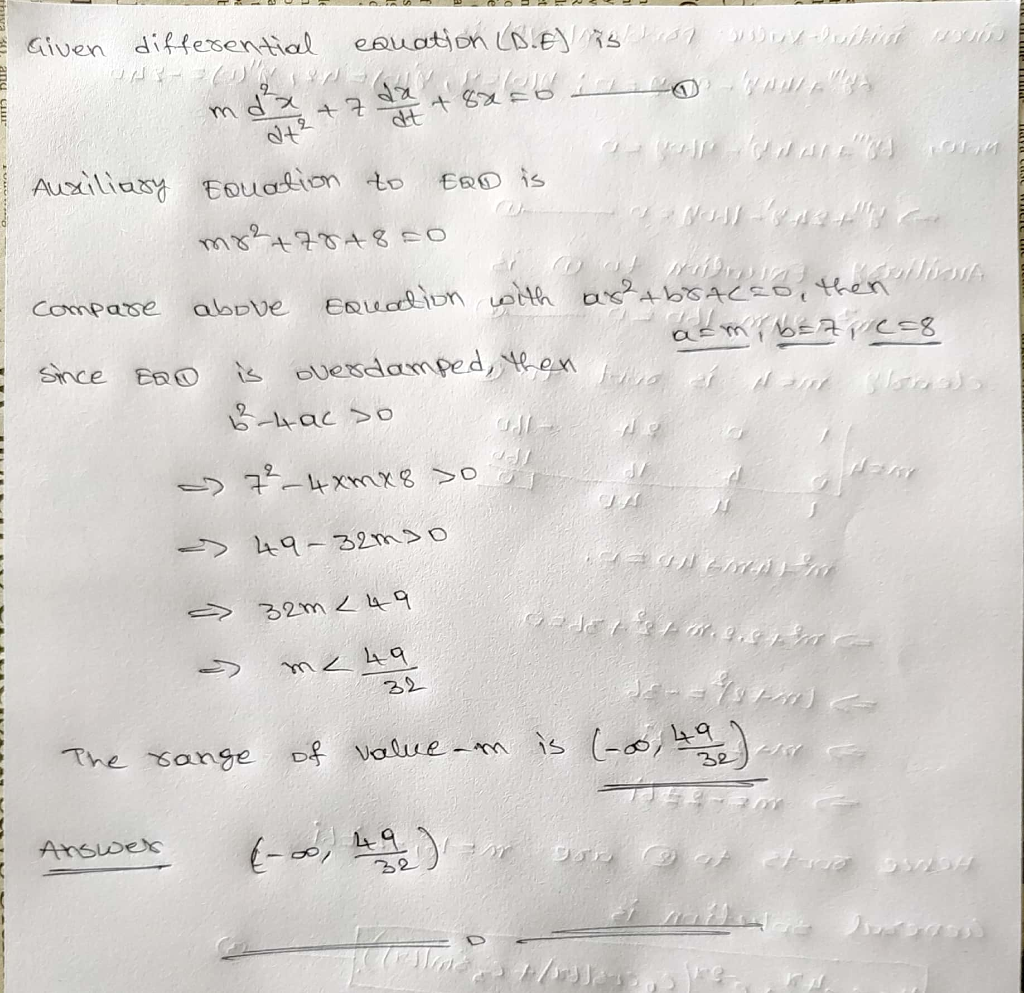##### Add Answer of: (1 point) If the differential equation d2x m 7 dx dt + 8x = 0 dt2...
Similar Homework Help Questions
• ### Problem 2. (1 point) If the differential equation dx m dt2 *sor + 8x = 0...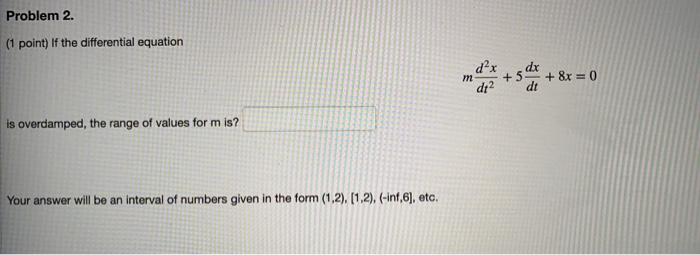Problem 2. (1 point) If the differential equation dx m dt2 *sor + 8x = 0 dt is overdamped, the range of values form is? Your answer will be an interval of numbers given in the form (1,2), (1,2), (-inf,6), etc.

• ### (1 point) If the differential equation m d dt2 da +4 dt + 6x = 0...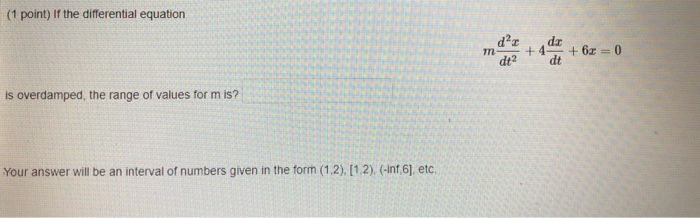(1 point) If the differential equation m d dt2 da +4 dt + 6x = 0 is overdamped, the range of values form is? Your answer will be an interval of numbers given in the form (1.2), (1.2) (-inf 6), etc.

• ### I AM REALLY STRUGGLING ON THIS PROBLEM PLEASE HELP ME CORRECT AND NEAT WORK IS MUCH...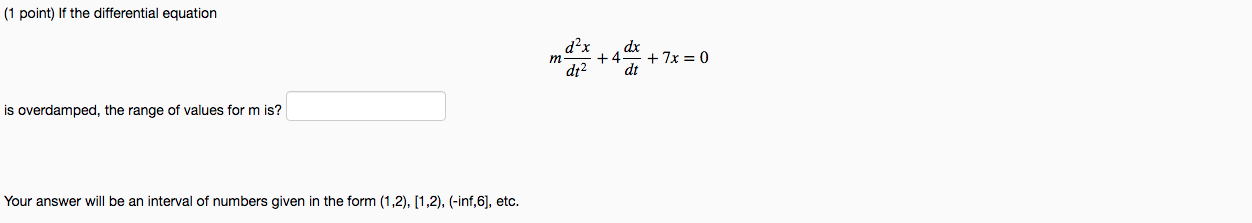I AM REALLY STRUGGLING ON THIS PROBLEM PLEASE HELP ME CORRECT AND NEAT WORK IS MUCH APPRECIATED THANKS (1 point) If the differential equation m dx +4 dt + 7x = 0 dt2 is overdamped, the range of values for m is? Your answer will be an interval of numbers given in the form (1,2), (1,2), (-inf,6), etc.

• ### STRUGGLING WITH THESE TWO SETS OF PROBLEMS ID APPREACIATE THE CORRECT ANSWERS THANKS (1 point) If...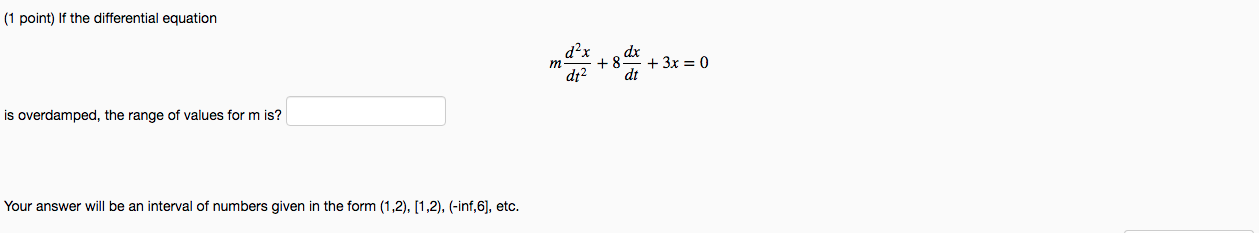STRUGGLING WITH THESE TWO SETS OF PROBLEMS ID APPREACIATE THE CORRECT ANSWERS THANKS (1 point) If the differential equation m dax + 8 dx dt2 dt + 3x = 0 is overdamped, the range of values form is? Your answer will be an interval of numbers given in the form (1,2), (1,2), (-inf,6), etc. (1 point) Find y as a function of t if y" - 6y + 8y = 0, y(O) = 5, y(1) = 4. yt) = Remark:...

• ### Problem 2. (1 point) If the differential equation de + 3x = 0 is overdamped, the...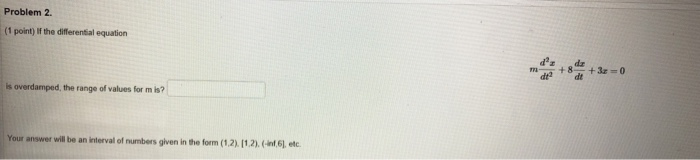Problem 2. (1 point) If the differential equation de + 3x = 0 is overdamped, the range of values for mis? Your answer will be an interval of numbers given in the form (1.2) 11.2).(-6), etc.

• ### For the system described by the following differential equation d3y(t) d2y(t) d2x(t) dy(t) 3 dt dx(t)...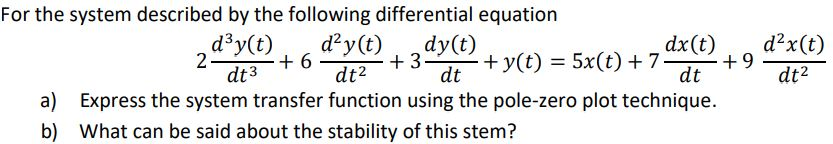For the system described by the following differential equation d3y(t) d2y(t) d2x(t) dy(t) 3 dt dx(t) 9 dt y(t) 5x(t) 7 2 6 dt3 dt2 dt2 Express the system transfer function using the pole-zero plot technique a) b) What can be said about the stability of this stem? For the system described by the following differential equation d3y(t) d2y(t) d2x(t) dy(t) 3 dt dx(t) 9 dt y(t) 5x(t) 7 2 6 dt3 dt2 dt2 Express the system transfer function using...

• ### (1 point) a. Consider the differential equation: d2y 0.16y-0 dt2 with initial conditions dt (0)-3 y(0)--1 and Find the...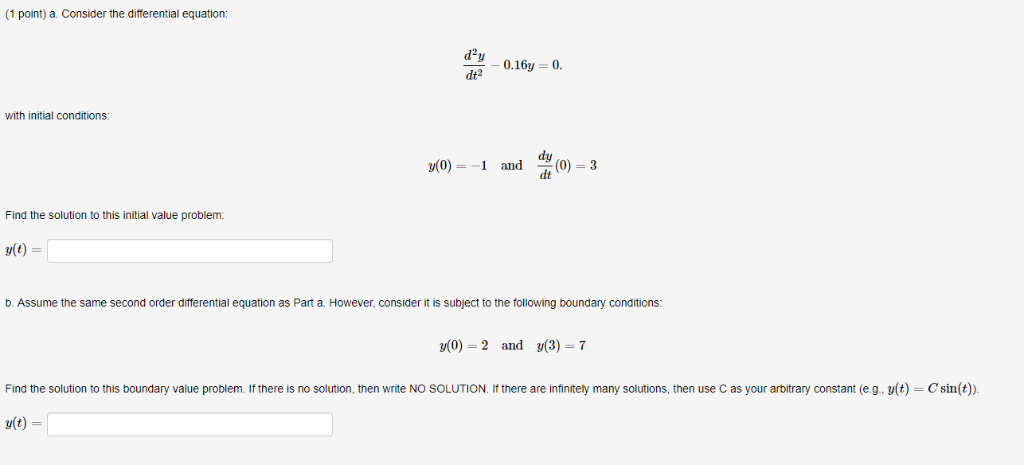(1 point) a. Consider the differential equation: d2y 0.16y-0 dt2 with initial conditions dt (0)-3 y(0)--1 and Find the solution to this initial value problem b. Assume the same second order differential equation as Part a. However, consider it is subject to the following boundary conditions: y(0)-2 and y(3)-7 Find the solution to this boundary value problem. If there is no solution, then write NO SOLUTION. If there are infinitely many solutions, then use C as your arbitrary constant (e.g....

• ### . 3- For this differential equation findy(t) if w( d 2w dı3 dt2 畿(0-)=-1 d2y dt2 (0-)=2 dt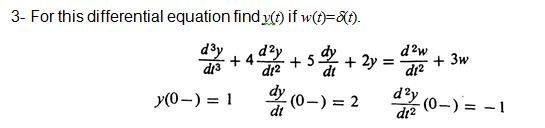Do not use Laplace transform methods to solve these problems. . 3- For this differential equation findy(t) if w( d 2w dı3 dt2 畿(0-)=-1 d2y dt2 (0-)=2 dt . 3- For this differential equation findy(t) if w( d 2w dı3 dt2 畿(0-)=-1 d2y dt2 (0-)=2 dt

• ### Rer Your answer will be an interval of numbers given in the form (1,2), (1,2), (-inf,6],...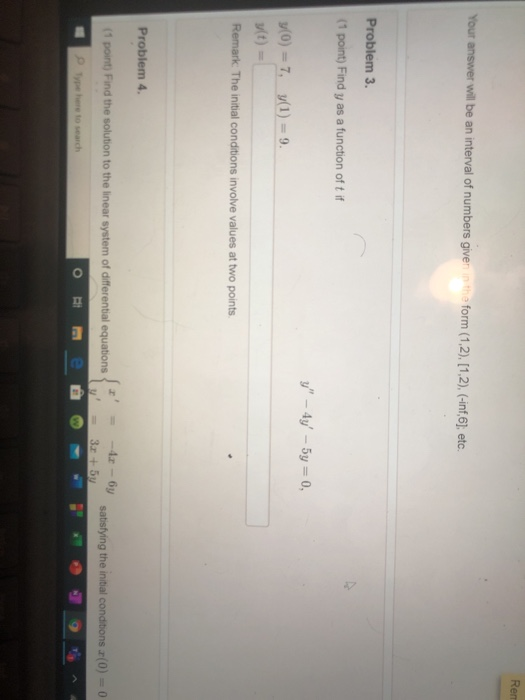Rer Your answer will be an interval of numbers given in the form (1,2), (1,2), (-inf,6], etc. Problem 3. (1 point) Find y as a function of t if y" – 4y' - 5y = 0, y(0) = 7, 3(1) = 9. u(t) Remark. The initial conditions involve values at two points. Problem 4. (1 point) Find the solution to the linear system of differential equations -42 - by 3.5 satisfying the initial conditions (0) = 0 Type here to...

• ### general solutions to the differential equation and plot. need help!

a. Use the general solutions x(t) to the differential equation d2x/dt2+2β*dx/dt+w02x=0 for underdamped, criticallydamped, and overdamped motion and choose the constants of integration to satisfy the initial conditions x=x0, and v=v0=0 at t=0.b. Use a computer to plot the results for x(t)/x0 as a function of w0t in the three cases β=(1/2)ω0,β=ω0, andβ=2ω0. show all three curves on a single plot.

Free Homework App